图形界面系列教材 （三）- Swing 的容器 JFrame和JDialog 图形界面系列教材 （五）- Swing 中的组件列表6分2秒

## 绝对定位

package gui; import javax.swing.JButton; import javax.swing.JFrame; public class TestGUI { public static void main(String[] args) { JFrame f = new JFrame("LoL"); f.setSize(400, 300); f.setLocation(200, 200); // 设置布局器为null，即进行绝对定位，容器上的组件都需要指定位置和大小 f.setLayout(null); JButton b1 = new JButton("英雄1"); // 指定位置和大小 b1.setBounds(50, 50, 80, 30); JButton b2 = new JButton("英雄2"); b2.setBounds(150, 50, 80, 30); JButton b3 = new JButton("英雄3"); b3.setBounds(250, 50, 80, 30); // 没有指定位置和大小，不会出现在容器上 JButton b4 = new JButton("英雄3"); f.add(b1); f.add(b2); f.add(b3); // b4没有指定位置和大小，不会出现在容器上 f.add(b4); f.setDefaultCloseOperation(JFrame.EXIT_ON_CLOSE); f.setVisible(true); } }

## FlowLayout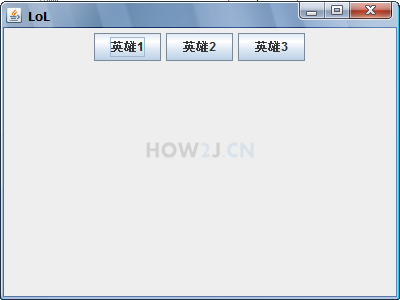package gui; import java.awt.FlowLayout; import javax.swing.JButton; import javax.swing.JFrame; public class TestGUI { public static void main(String[] args) { JFrame f = new JFrame("LoL"); f.setSize(400, 300); f.setLocation(200, 200); // 设置布局器为FlowLayerout // 容器上的组件水平摆放 f.setLayout(new FlowLayout()); JButton b1 = new JButton("英雄1"); JButton b2 = new JButton("英雄2"); JButton b3 = new JButton("英雄3"); // 加入到容器即可，无需单独指定大小和位置 f.add(b1); f.add(b2); f.add(b3); f.setDefaultCloseOperation(JFrame.EXIT_ON_CLOSE); f.setVisible(true); } }

## BorderLayout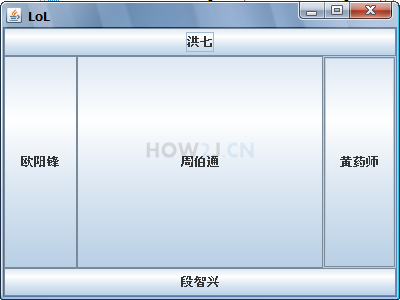package gui; import java.awt.BorderLayout; import java.awt.FlowLayout; import javax.swing.JButton; import javax.swing.JFrame; public class TestGUI { public static void main(String[] args) { JFrame f = new JFrame("LoL"); f.setSize(400, 300); f.setLocation(200, 200); // 设置布局器为BorderLayerout // 容器上的组件按照上北下南左西右东中的顺序摆放 f.setLayout(new BorderLayout()); JButton b1 = new JButton("洪七"); JButton b2 = new JButton("段智兴"); JButton b3 = new JButton("欧阳锋"); JButton b4 = new JButton("黄药师"); JButton b5 = new JButton("周伯通"); // 加入到容器的时候，需要指定位置 f.add(b1, BorderLayout.NORTH); f.add(b2, BorderLayout.SOUTH); f.add(b3, BorderLayout.WEST); f.add(b4, BorderLayout.EAST); f.add(b5, BorderLayout.CENTER); f.setDefaultCloseOperation(JFrame.EXIT_ON_CLOSE); f.setVisible(true); } }

## GridLayout

GridLayout，即网格布局器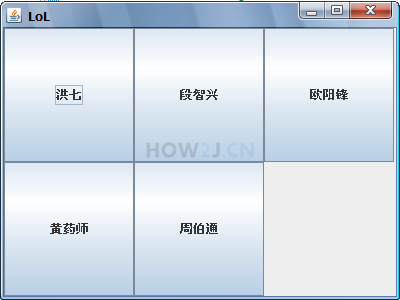package gui; import java.awt.GridLayout; import javax.swing.JButton; import javax.swing.JFrame; public class TestGUI { public static void main(String[] args) { JFrame f = new JFrame("LoL"); f.setSize(400, 300); f.setLocation(200, 200); // 设置布局器为GridLayerout，即网格布局器 // 该GridLayerout的构造方法表示该网格是2行3列 f.setLayout(new GridLayout(2, 3)); JButton b1 = new JButton("洪七"); JButton b2 = new JButton("段智兴"); JButton b3 = new JButton("欧阳锋"); JButton b4 = new JButton("黄药师"); JButton b5 = new JButton("周伯通"); f.add(b1); f.add(b2); f.add(b3); f.add(b4); f.add(b5); f.setDefaultCloseOperation(JFrame.EXIT_ON_CLOSE); f.setVisible(true); } }

## setPreferredSize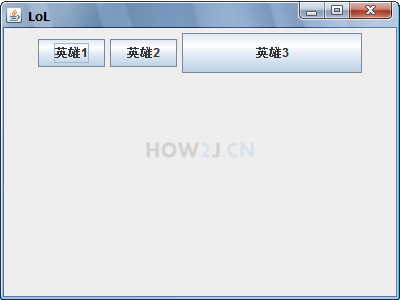package gui; import java.awt.Dimension; import java.awt.FlowLayout; import javax.swing.JButton; import javax.swing.JFrame; public class TestGUI { public static void main(String[] args) { JFrame f = new JFrame("LoL"); f.setSize(400, 300); f.setLocation(200, 200); f.setLayout(new FlowLayout()); JButton b1 = new JButton("英雄1"); JButton b2 = new JButton("英雄2"); JButton b3 = new JButton("英雄3"); // 即便 使用 布局器 ，也可以 通过setPreferredSize，向布局器建议该组件显示的大小 b3.setPreferredSize(new Dimension(180, 40)); f.add(b1); f.add(b2); f.add(b3); f.setDefaultCloseOperation(JFrame.EXIT_ON_CLOSE); f.setVisible(true); } }

## CardLayout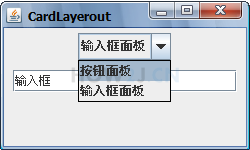## 练习-计算器上的按钮

姿势不对,事倍功半! 点击查看做练习的正确姿势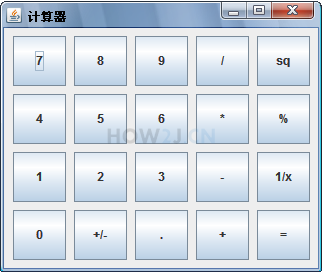## 答案-计算器上的按钮2分11秒 本视频采用html5方式播放，如无法正常播放，请将浏览器升级至最新版本，推荐火狐，chrome，360浏览器。 如果装有迅雷，播放视频呈现直接下载状态，请调整 迅雷系统设置-基本设置-启动-监视全部浏览器 (去掉这个选项)。 chrome 的 视频下载插件会影响播放，如 IDM 等，请关闭或者切换其他浏览器

 图形界面系列教材 （三）- Swing 的容器 JFrame和JDialog 图形界面系列教材 （五）- Swing 中的组件列表

HOW2J公众号，关注后实时获知最新的教程和优惠活动，谢谢。2021-10-16 练习 - 简化版计算器
 莫諾 关于 JAVA 中级-图形界面-布局器 的提问 使用布局器做出计算器上的按钮效果```JFrame f = new JFrame("简化版计算器"); f.setSize(300, 265); f.setLocation(200, 200); f.setLayout(new FlowLayout()); JButton b1 = new JButton("7"); JButton b2 = new JButton("8"); JButton b3 = new JButton("9"); JButton b4 = new JButton("/"); JButton b5 = new JButton("sq"); JButton b6 = new JButton("4"); JButton b7 = new JButton("5"); JButton b8 = new JButton("6"); JButton b9 = new JButton("*"); JButton b10 = new JButton("%"); JButton b11 = new JButton("1"); JButton b12 = new JButton("2"); JButton b13 = new JButton("3"); JButton b14 = new JButton("-"); JButton b15 = new JButton("1/x"); JButton b16 = new JButton("0"); JButton b17 = new JButton("+/-"); JButton b18 = new JButton("."); JButton b19 = new JButton("+"); JButton b20 = new JButton("="); b1.setPreferredSize(new Dimension(51, 51));b2.setPreferredSize(new Dimension(51, 51));b3.setPreferredSize(new Dimension(51, 51));b4.setPreferredSize(new Dimension(51, 51));b5.setPreferredSize(new Dimension(51, 51)); b6.setPreferredSize(new Dimension(51, 51));b7.setPreferredSize(new Dimension(51, 51));b8.setPreferredSize(new Dimension(51, 51));b9.setPreferredSize(new Dimension(51, 51));b10.setPreferredSize(new Dimension(51, 51)); b11.setPreferredSize(new Dimension(51, 51));b12.setPreferredSize(new Dimension(51, 51));b13.setPreferredSize(new Dimension(51, 51));b14.setPreferredSize(new Dimension(51, 51));b15.setPreferredSize(new Dimension(51, 51)); b16.setPreferredSize(new Dimension(51, 51));b17.setPreferredSize(new Dimension(51, 51));b18.setPreferredSize(new Dimension(51, 51));b19.setPreferredSize(new Dimension(51, 51));b20.setPreferredSize(new Dimension(51, 51)); f.add(b1);f.add(b2);f.add(b3);f.add(b4);f.add(b5); f.add(b6);f.add(b7);f.add(b8);f.add(b9);f.add(b10); f.add(b11);f.add(b12);f.add(b13);f.add(b14);f.add(b15); f.add(b16);f.add(b17);f.add(b18);f.add(b19);f.add(b20); f.setDefaultCloseOperation(JFrame.EXIT_ON_CLOSE); f.setVisible(true); ```

1 个答案

First_Auraro

2021-04-13 有什么方法可以竖直排列吗？
 byxdbyj玉 关于 JAVA 中级-图形界面-布局器 的提问 有什么方法可以竖直排列吗？

2020-05-02 计算器
2020-02-23 简单版
2019-04-06 那个中间模板感觉看着有点别扭